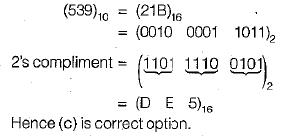Courses

# Test: Number System- 1

## 10 Questions MCQ Test Question Bank for GATE Computer Science Engineering | Test: Number System- 1

Description
This mock test of Test: Number System- 1 for Computer Science Engineering (CSE) helps you for every Computer Science Engineering (CSE) entrance exam. This contains 10 Multiple Choice Questions for Computer Science Engineering (CSE) Test: Number System- 1 (mcq) to study with solutions a complete question bank. The solved questions answers in this Test: Number System- 1 quiz give you a good mix of easy questions and tough questions. Computer Science Engineering (CSE) students definitely take this Test: Number System- 1 exercise for a better result in the exam. You can find other Test: Number System- 1 extra questions, long questions & short questions for Computer Science Engineering (CSE) on EduRev as well by searching above.
QUESTION: 1

Solution:
QUESTION: 2

Solution:
QUESTION: 3

### Why are digital circuits easier to design than analog circuits?

Solution:
QUESTION: 4

A digital gate can respond to an input signal in

Solution:
QUESTION: 5

Analog methods are not used for handling extremely precise information because

Solution:
QUESTION: 6

What values of A, B, C and D satisfy the following simultaneous boolean equation?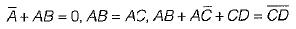1. A = 1. B = 0, C = 0, D = 1
2. A = 1, B = 1, C= 0, D = 0
3. A = 1, B = 0, C = 1, D = 1
4. A = 1, B = 0, C = 0, D = 0

Solution: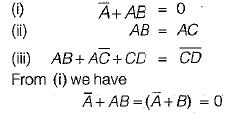i.e. A must be 1 and B must be 0 From (ii) we have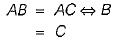i.e. C is also 0.
Putting the values of A, B and C in (iii)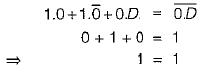For, the above equation to be true D is independent.

QUESTION: 7

The number of 1s in the binary representation of (3* 4096 + 15*256 + 5* 16 + 3) are

Solution:

3*4096 + 15*256 + 5*16 + 3
As we can see that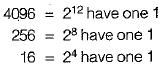Hence, they only remains as same in number of 1’s and when any other number is multiplied by themselves, the number of 1 ’s is only the count of the number of 1 ’s in other multiplicant.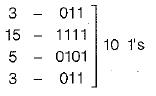∴ correct option is (c)

QUESTION: 8

Which of the following sets of components is/are sufficient to implement any arbitrary boolean function:
1. XOR gates, NOT gates
2. 2 to 1 multiplexers
3. AND gates, XOR gates
4. Three-input gates that output (A • B) + C for the inputs A . B. and C.

Solution: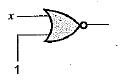2 to 1 multiplexer is a universal circuit.

QUESTION: 9

Zero has two representation in

Solution:
QUESTION: 10

The 2’s complement representation of (539)10 in hexadecimal is

Solution: